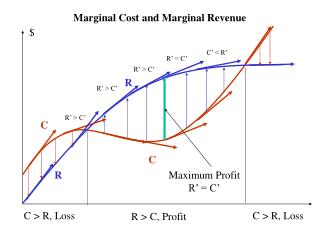# Marginal Cost and Marginal Revenue - PowerPoint PPT PresentationDownload PresentationMarginal Cost and Marginal Revenue

Marginal Cost and Marginal RevenueDownload Presentation## Marginal Cost and Marginal Revenue

- - - - - - - - - - - - - - - - - - - - - - - - - - - E N D - - - - - - - - - - - - - - - - - - - - - - - - - - -
##### Presentation Transcript

1. Marginal Cost and Marginal Revenue \$ C’ < R’ R’ = C’ R’ > C’ R R’ > C’ R’ > C’ C C R Maximum ProfitR’ = C’ C > R, Loss C > R, Loss R > C, Profit

2. \$ R C C Max Profit, P’ =0 then R’ = C’ R P=R-C Break Even, P =0 then R = C Cost, Revenue and Profit R > C, Profit C > R, Loss C > R, Loss